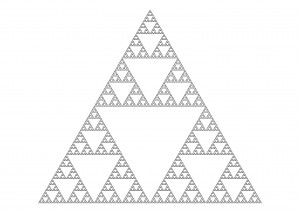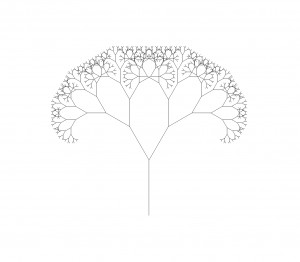# Recursions and L-System

back
Design with recursions and L-System pattern.
Chen, 2016-01-26

It is actually very easy if you understand and know how to use recursive function in Python. You can basically let the function run again and again inside the function by itself just with a condition statement.

For example, a Fibonacci Sequence is a recursive sequence that looks like this:

`0,1,1,2,3,5,8,13,21,34,55,89,144…`

the first two numbers are 0 and 1. Then the following numbers apply to a very simple rule which is:

`F(n) = F(n-1) + F(n-2)`

So in python you can code like this:

``````def fibo(i):
if i == 0 : return 0
if i == 1 : return 1
else:
return fibo(i-1)+fibo(i-2)

for i in range(10):
print fibo(i)
``````

If you understand that, the recursive process below would be much easier to follow:

#### demo 1

``````import rhinoscriptsyntax as rs

def recursiveExample():
rs.EnableRedraw(False)
triangle = rs.GetObject("select a triangle",4)
gen = 6
subtriangle(triangle,gen)

def subtriangle(triangle,maxGen):
vertexPts = rs.PolylineVertices(triangle)
m0 = (vertexPts+vertexPts)/2
m1 = (vertexPts+vertexPts)/2
m2 = (vertexPts+vertexPts)/2

if maxGen == 0:
return
else:
subtriangle(triangle0,maxGen-1)
subtriangle(triangle1,maxGen-1)
subtriangle(triangle2,maxGen-1)

if __name__ == "__main__" : recursiveExample()
``````#### demo 2

``````import rhinoscriptsyntax as rs
rs.EnableRedraw(False)

def main():
line = rs.GetObject("select a branch",4)
gen = 8
scale = 0.7
angle = 30
branching(line,gen,scale,angle)

def branching(line,maxGen,scale,angle):

startPt = rs.CurveStartPoint(line)
endPt = rs.CurveEndPoint(line)

vec = rs.VectorCreate(endPt,startPt)
vec = rs.VectorScale(vec,scale)
axis = [0,0,1]
vec0 = rs.VectorRotate(vec,angle,axis)
vec1 = rs.VectorRotate(vec,-angle,axis)